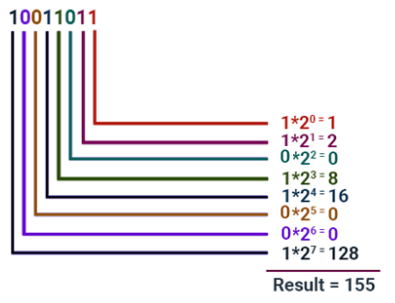# Binary to Decimal Formula

Converting binary to a decimal number or decimal to binary number is an easy task. But, you need to be careful not to mix up the two sets of numbers. For instance, if you write the digits 10 on the page it could mean the number “ten” if we assume it to be a decimal number, or it could equally be a “1” and a “0” together in binary, which is equal to the number two in the weighted decimal format from above table.
$\large Decimal Number = n^{th}bit \times 2^{n-1}$

Binary to Decimal Formula:

$\large N = b_{n}q^{n} + b_{n-1}q^{n-2} + ….. + b_{2}q^{2} + b_{1}q^{1} + b_{0}q^{0} + b_{-1}q^{-1} + b_{-2}q^{-2}$
Where,
N is decimal equivalent,
b is the digit,
q is the base value that starts from the most significant digit order qn to least significant order q-1, q-2, …..

To convert binary to decimal the following chart is used and binary is noted as per the given decimal number.

 Binary 0 1 10 11 100 101 110 111 1000 1001 1010 1011 1100 1101 1110 1111 Decimal 0 1 2 3 4 5 6 7 8 9 10 11 12 13 14 15

So, for instance, if you use a binary number string it should add the subscript “2” to denote a base 2 number so the binary number would be written as

$$\begin{array}{l}10_{2}\end{array}$$
. Likewise, if it was a standard decimal number it would add the subscript “10” to denote a base 10 and written as
$$\begin{array}{l}10_{10}\end{array}$$
.To convert binary into decimal is very simple and can be done as shown below:
Say we want to convert the 8 bit value 10011101 into a decimal value, we can use a formula table like that below:
 128 64 32 16 8 4 2 1 1 0 0 1 1 1 0 1

To convert, you simply take a value from the top row wherever there is a 1 below and then add the values together.

This will give 157 as the result.

### Solved Examples

Question 1: Convert 0110101 to decimal.
Solution:
Given Binary number is 0110101
0110101 = (0
$$\begin{array}{l}\times\end{array}$$
$$\begin{array}{l}2^{6}\end{array}$$
) + (1
$$\begin{array}{l}\times\end{array}$$
$$\begin{array}{l}2^{5}\end{array}$$
) + (1
$$\begin{array}{l}\times\end{array}$$

$$\begin{array}{l}2^{4}\end{array}$$
) + (0
$$\begin{array}{l}\times\end{array}$$
$$\begin{array}{l}2^{3}\end{array}$$
) + (1
$$\begin{array}{l}\times\end{array}$$
$$\begin{array}{l}2^{2}\end{array}$$
) + (0
$$\begin{array}{l}\times\end{array}$$
$$\begin{array}{l}2^{1}\end{array}$$
) + (1
$$\begin{array}{l}\times\end{array}$$
$$\begin{array}{l}2^{0}\end{array}$$
)
= 0 + 32 + 16 + 0 + 4 + 0 + 1
= 53
Therefore, Binary Number 0110101 = 53 Decimal number

Question 2: Convert the binary number 10100011 to decimal.

Solution:

Given binary number is 10100011

Using the conversion formula,

10100011 = (1 × 27) + (0 × 26) + (1 × 25) + (0 × 24) + (0 × 23) + (0 × 22) + (1 × 21) + (1 × 20)

= 128 + 0 + 32 + 0 + 0 + 0 + 2 + 1

= 163

Therefore, binary number 10100011 = 163 decimal number

Question 3: Convert the binary number 11101111 to decimal.

Solution:

Given binary number is 11101111

Using the conversion formula,

11101111 = (1 × 27) + (1 × 26) + (1 × 25) + (0 × 24) + (1 × 23) + (1 × 22) + (1 × 21) + (1 × 20)

= 128 + 64 + 32 + 0 + 8 + 4 + 2 + 1

= 239

Therefore, binary number 11101111 = 239 decimal number